Next: Dynamic Programming Approach Up: Appendix Previous: Appendix

## Search Space and Error Analysis

For a tree with n different leaves, there are in the order of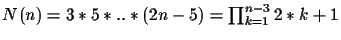different tree topologies . If a circular order is given, there are O(2n-3) different trees that can be built with that order. So a circular order alone reduces the search space. To get an intuition we build a small table (see Table 7.1).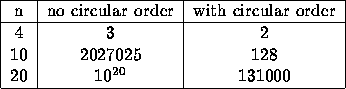We have seen that the probability to get a wrong TSP order is very small. But given a correct TSP order, there are still 2n-3 possible different trees. So far we know that if the error of each distance measurement is not larger then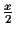, where x is the shortest edge length, we can guarantee that the tree construction is correct. As a next step we want to determine the probability to get a wrong tree for a given normal distribution of the distance measurement. Our goal is to express the probability to get a wrong edge in terms of the shortest edge x. At each connection step, we connect leaf si and si+1 with the smallest. Now assume thathas some error s.t we connect the wrong leaves, and one of the correct choices would have been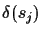. We know that if there was no error, then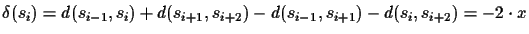We assume that the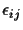follow a normal distribution with standard deviation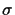. Then the error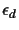also follows a normal distribution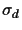, where the standard deviation is the sum of the individual standard deviations: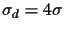(see Figure 12). In order to get a wrong connection, the following condition must be true: for all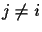, whereis a correct connection,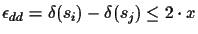. This again is a normal distribution, with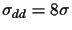.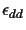must be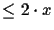for all possible valid connections. For a balanced tree with n leaves (tree depth log(n)), there are at most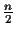valid connections. In the worst case, if the tree is extremely unbalanced, there is only 1 correct connection at each step. Let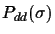be the probability that one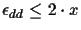for a given standard deviation: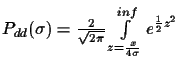So the overall probability to get a wrong connection is: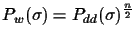in the best case, and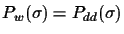in the worst case.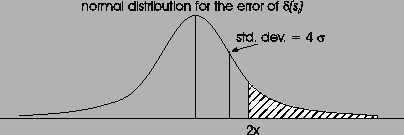To illustrate the probability to get the shortest edge x wrong we build a table (see Table 14) for an example tree of 16 leaves. So if the standard deviationof the errorof the distance measurement is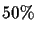of the shortest edge x, then the probability to get this edge wrong is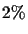in the best, and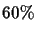in the worst case. Hence for very large errors, a dynamic programming approach is more appropriate.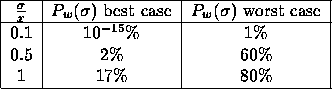Next: Dynamic Programming Approach Up: Appendix Previous: Appendix
Chantal Korostensky
1999-07-14